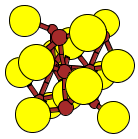Crystal Lattice Structures: Creation Date:  3 May 2002 Last Modified: 21 Oct 2004

# The Ni3S2 (D5e) StructureYou can now

• This can be considered as a prototype for a high concentration of ordered vacancies in the hcp structure. We get the ideal hcp atomic positions when y = 1/6 and z = 1/3, leaving a vacancy at the origin

• Prototype: Ni3S2
• Pearson Symbol: hR5
• Strukturbericht Designation: D5e
• Space Group: R32 (Cartesian and lattice coordinate listings available)
• Number: 155
• Other Compounds with this Structure: Ni3Se2
• Primitive Vectors:

The rhombohedral lattice can be specified by the length of the primitive vectors, a, and the angle between them, θ, or by the lattice constants of the equivalent hexagonal lattice. In terms of a and θ we have

ahex = 2 a sin(½ θ)

chex = a {3 [1 + 2 cos(θ)]}½

Pearsons' Handbook gives the lattice parameters for the hexagonal lattice. In rhombohedral form:

 A1 = + ½ ahex X - 12-½ ahex Y + 1/3 chex Z A2 = + 3-½ ahex Y + 1/3 chex Z A3 = - ½ ahex X - 12-½ ahex Y + 1/3 chex Z
• Basis Vectors:

Pearson's Handbook gives the coordinates of the atoms in the hexagonal unit cell. In terms of the rhombohderal cell, we have

zrhomb = zhex       &       yrhomb = xhex - ½

 B1 = + z A1 + z A2 + z A3 = + z c Z (2c) (S) B2 = - z A1 - z A2 - z A3 = - z c Z (2c) (S) B3 = ½ A1 + y A2 - y A3 = ¼ (2 y + 1) a X + 48-½ (6 y - 1) a Y + 1/6 c Z (3e) (Ni) B4 = - y A1 + ½ A2 + y A3 = - y a X + 12-½ a Y + 1/6 c Z (3e) (Ni) B5 = + y A1 - y A2 + ½ A3 = ¼ (2 y - 1) a X - 48-½ (6 y + 1) a Y + 1/6 c Z (3e) (Ni)

Go back to the Sulfur and its compounds page.

Go back to Crystal Lattice Structure page.

 Structures indexed by: This is a mirror of an old page created at theNaval Research LaboratoryCenter for Computational Materials ScienceThe maintained successor is hosted at http://www.aflowlib.org/CrystalDatabase/ and published as M. Mehl et al., Comput. Mater. Sci. 136 (Supp.), S1-S828 (2017).